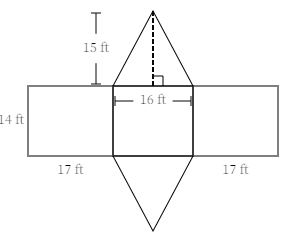ResourcesTestimonials
Plans
ResourcesTestimonials
Plans
Worksheet

# Find Surface Area Given A Net Of A Triangular Prism Worksheet

## 8 problems6th graders should understand the definition of a triangular prism and how to use its net to determine the surface area. Teachers can use a "Find surface area given a net of a triangular prism Worksheet" to help their students gain proficiency in this skill. The net of a triangular prism is the unfolded two-dimensional representation of the prism. By calculating the area of each of its faces and adding them together, students can determine the surface area of a triangular prism.

Geometry
6.G.A.4

For example: What is the surface area of the triangular prism?Solution:

The above net has 2 triangles and 3 rectangles.

Area of first triangle = ½*b*h = ½*16*15 = 120 ft^2

Area of second triangle = Area of first triangle = 120 ft^2

Area of left rectangle = l*w = 17*14 = 238 ft^2

Area of middle rectangle = l*w = 16*14 = 224 ft^2

Area of right rectangle = l*w = 17*14 = 238 ft^2

Surface area of the triangular prism = Composite area of 2 triangle and 3 rectangles

= 120 + 120 + 238 + 224 + 238

= 940

Therefore, the surface area of the triangular prism is 940 ft^2.

For example: What is the surface area of the triangular prism?Solution:

The above net has 2 triangles and 3 rectangles.

Area of first triangle = ½*b*h = ½*16*15 = 120 ft^2

Area of second triangle = Area of first triangle = 120 ft^2

Area of left rectangle = l*w = 17*14 = 238 ft^2

Area of middle rectangle = l*w = 16*14 = 224 ft^2

Area of right rectangle = l*w = 17*14 = 238 ft^2

Surface area of the triangular prism = Composite area of 2 triangle and 3 rectangles

...

Show all

### What teachers are saying about BytelearnWhat teachers are sayingStephen Abate
19-year math teacher
Carmel, CA
Any math teacher that I know would love to have access to ByteLearn.Jennifer Maschino
4-year math teacher
Summerville, SC
“I love that ByteLearn helps reduce a teacher’s workload and engages students through an interactive digital interface.”Rodolpho Loureiro
Dean, math program manager, principal
Miami, FL
“ByteLearn provides instant, customized feedback for students—a game-changer to the educational landscape.”